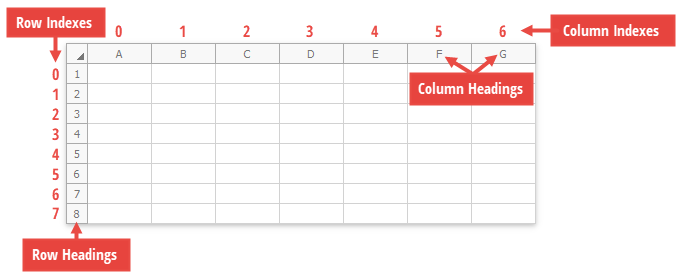.NET Framework 4.5.2+

# How to: Access a Row or Column

## Rows

This example demonstrates how to access rows in a worksheet. Use the Worksheet.Rows property to get a collection of rows contained in a worksheet (the RowCollection object). To get an individual row by its index (zero-based) or heading (“1”, “2”, “3”, etc.), use the RowCollection.Item property.

``````using DevExpress.Spreadsheet;
// ...

Workbook workbook = new Workbook();

// Access a collection of rows.
RowCollection rows = workbook.Worksheets.Rows;

// Access the first row by its index in the collection of rows.
Row firstRow_byIndex = rows;

// Access the first row by its unique name.
Row firstRow_byName = rows["1"];
``````

## Columns

This example demonstrates how to access columns in a worksheet. Use the Worksheet.Columns property to get a collection of columns contained in a worksheet (the ColumnCollection object). To get an individual column by its index (zero-based) or heading (“A”, “B”, “C”, etc.), use the ColumnCollection.Item property.

``````using DevExpress.Spreadsheet;
// ...

Workbook workbook = new Workbook();

// Access a collection of columns.
ColumnCollection columns = workbook.Worksheets.Columns;

// Access the first column by its index in the collection of columns.
Column firstColumn_byIndex = columns;

// Access the first column by its unique name.
Column firstColumn_byName = columns["A"];
``````

The image below shows row and column indexes and headings in a worksheet, when a workbook is opened in Microsoft® Excel®.